# L2-CAF: A Neural Network Debugger

Every software engineer has used a debugger to debug his code. Yet, a neural network debugger… That’s news! This paper  proposes a debugger to debug and visualize attention in convolutional neural networks (CNNs).

Before describing the CNN debugger, I want to highlight few attributes of program debuggers (e.g., gdb): (I) Debuggers are not program-specific, i.e., we use the same debugger to debug many programs; (II) We use a debugger to debug a few lines in our code, i.e., not every line; (III) Before using a debugger, we decide consciously which part of the program we want to debug (e.g., which function); this is where we set our breakpoints; (IV) A debugger helps us understand a program by pausing its execution at a breakpoint (e.g., S1), then executing a few instructions till another point (e.g., S2). By inspecting the key changes between S1 and S2, we understand our code. Figure 1 illustrates how a debugger helps us execute a few instructions between two points in our program: S1 & S2.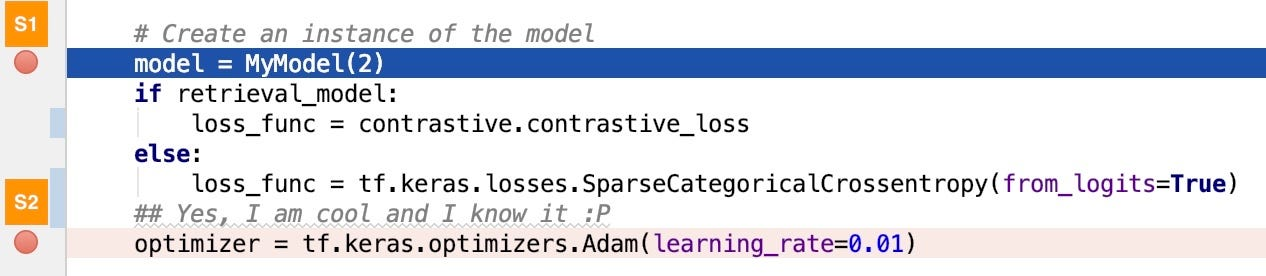Figure 1: We debug our code using a debugger (e.g., gdb). The debugger helps us execute a few instructions between two points in our programs (S1 and S2).

We tend to regard neural networks as black boxes. Can’t we design a debugger neural network to interpret these black boxes?! I believe this is possible. L2-CAF  is a tiny neural network — of size 7x7 — that can visualize attention in neural networks. L2-CAF (I) supports a large spectrum of architectures; (II) visualizes attention at both the last and intermediate convolutional layers, i.e., can debug various parts inside a given network; (III) Before using L2-CAF, we decide consciously which part of the network we want to debug (e.g., which layer); (IV) L2-CAF helps us understand a network by monitoring its execution between two points (S1 and S2), then identifying the key features between these two points. Figure 2 illustrates how L2-CAF debugs a CNN.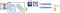Figure 2: L2-CAF debugs a CNN. L2-CAF helps us understand the CNN by both monitoring its execution between two points (S1 and S2) and identifying the key features between these two points.

Given a trained CNN as shown in Figure 2, L2-CAF feeds an input image x through a normal feed-forward pass (green solid path) to generate the network output NT(x). Then, L2-CAF feeds x again but multiplies the last convolutional feature map by a filter f (orange dashed path) to generate a new filtered output FT(x,f). L2-CAF optimizes f using gradient descent to minimize the difference between NT(x) and FT(x, f) while constraining the L2-Norm of the filter f.

Formally speaking, L2-CAF casts attention visualization as a constrained optimization problem. It leverages the L2-Norm Constraints as an Attention Filter, and hence the name L2-CAF. L2-CAF solves the following constraint optimization problem using gradient descent. For those comfortable with optimization problems, the following equation should be familiar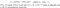Figure 3: L2-CAF solves this constraint optimization problem to visualize attention in CNNs. L2-CAF is a tiny debugger network that debugs CNNs.

Figure 4 depicts the Hadamard (element-wise) multiplication between the convolutional feature maps and the L2-CAF. In standard architectures, the last convolutional feature maps A has K channels and 7x7 spatial dimensions. Accordingly, L2-CAF is a filter with a size of 7x7. If L2-CAF is all ones, the objective function ||NT(x)-FT(x,f)|| equals zero, but the L2-Norm constraint is violated — ||f||_2 = sqrt(49) = 7. In contrast, If L2-CAF identifies the key features in A, the L2-Norm constraint is satisfied (||f||_2=1) and ||NT(x)-FT(x,f)|| is minimum.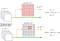Figure 4: L2-CAF multiplies the convolutional feature maps by the filter f using Hadamard (element-wise) multiplication. (Top) The filter f is all ones, the objective function equals zero but the L2-Norm constraint is violated. (Bottom) The filter highlights a key feature inside the feature maps; accordingly, the objective function is minimum while the L2-Norm constraint is satisfied.

To debug a CNN, L2-CAF leverages gradient descent to identify the key features inside the feature maps. Gradient descent guides L2-CAF to find the optimal filter f that minimizes the loss function ||NT(x)-FT(x,f)|| while satisfying the L2-Norm constraint. The L2-Norm constraint has two benefits. The first benefit is that it avoids a trivial solution where L2-CAF converges to all ones and concludes that all features are equally important as shown in Figure 4 (Top).

The second benefit of the L2-Norm constraint is that it supports multiple modes. Accordingly, L2-CAF can attend to multiple locations at the same time. That is important when an image contains multiple objects at different locations as shown in Figure 5.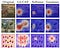Figure 5: L2-CAF supports other constraints. Yet, the L2-Norm constraint is the best. A softmax constraint offers a sparse result while the Gaussian filter assumes a single mode. L2-CAF supports multi-modes.

The L2-CAF network comes in two different flavors: class-oblivious and class-specific. The class-oblivious L2-CAF is best for feature embedding networks (e.g., retrieval and representation learning networks). These networks output a feature embedding with d dimensionality where each dimension represents an aspect of the network input. In contrast, the class-specific L2-CAF is best for classification networks. The class-specific L2-CAF can generate a heatmap for each output logit (different classes) making it perfect for images with multiple distinct objects as shown in Figure 6.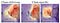Figure 6: L2-CAF comes in two flavors: class-oblivious and class-specific. This separates L2-CAF from dominant literature that targets classification networks only.

When debugging our code, we can set our breakpoints at any point. Similarly, L2-CAF can set its breakpoints at different parts of the network. Accordingly, L2-CAF visualizes attention in both the last and intermediate convolutional layers. Figure 7 shows how L2-CAF can visualize two objects simultaneously in a given image. L2-CAF delivers coarse-grained attention when applied on the last conv layer, and fine-grained attention when applied on an intermediate conv layer.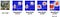Figure 7: L2-CAF visualizes attention in both the last and intermediate convolutional layers.

Finally, I hope this article has delivered the following key point: We can understand a neural network by debugging it, like the way we debug our source code. L2-CAF is the first neural network debugger, but further extensions are required.

References

 Taha, A., Yang, X., Shrivastava, A. and Davis, L. A Generic Visualization Approach for Convolutional Neural Networks. ECCV 2020.

Tensorflow Implementation

PyTorch Implementation

I write reviews on computer vision papers. Writing tips are welcomed.

## More from Ahmed Taha

I write reviews on computer vision papers. Writing tips are welcomed.